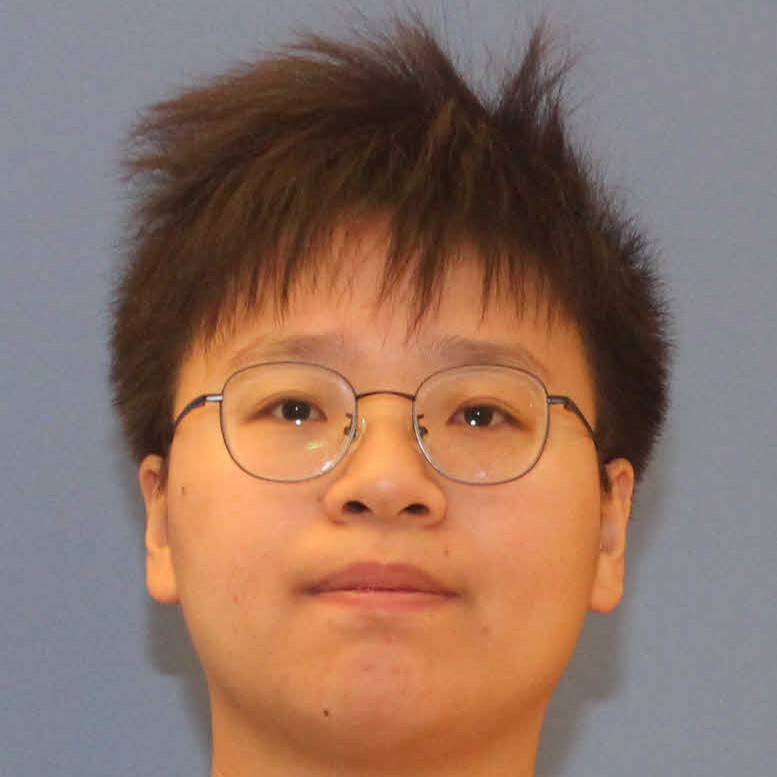#### Home

•### Large scale geometry of graphs of polynomial growth

###### Speaker

Jing Yu, Georgia Institute of Technology###### Time

2023-08-28 13:30:00 ~ 2023-08-28 14:30:00

###### Abstract

In 1995, Levin and Linial, London, and Rabinovich conjectured that every connected graph G of polynomial growth admits an injective homomorphism to the n-dimensional grid graph for some n. Moreover, they conjectured that if every ball of radius r in G contains at most O(r^𝜌) vertices, then one can take n = O(𝜌). Krauthgamer and Lee confirmed the first part of this conjecture and refuted the second in 2007. Prompted by these results, Papasoglu asked whether a graph G of polynomial growth admits a coarse embedding into a grid graph. We give an affirmative answer to this question. Moreover, it turns out that the dimension of the grid graph only needs to be linear in the asymptotic growth rate of G, which confirms the original Levin-Linial-London-Rabinovich conjecture *in the asymptotic sense*. All our results are proved for Borel graphs, which allows us to settle a number of problems in descriptive combinatorics. Roughly, we prove that graphs generated by free Borel actions of ℤⁿ are universal for the class of Borel graphs of polynomial growth. This provides a general method for extending results about ℤⁿ-actions to all Borel graphs of polynomial growth. For example, an immediate consequence of our main result is that all Borel graphs of polynomial growth are hyperfinite, which answers a well-known question in the area. Along the way we find an alternative, probabilistic proof of a theorem of Papasoglu that graphs of asymptotic polynomial growth rate 𝜌<∞ have asymptotic dimension at most 𝜌 and establish the same bound in the Borel setting. This is joint work with Anton Bernshteyn. This is joint work with Anton Bernshteyn.

###### Bio

Jing Yu is a PhD candidate in ACO at Georgia Institute of Technology. Her research interest lies in combinatorics and descriptive set theory. She obtained her BS and MS in mathematics from Fudan University.

© John Hopcroft Center for Computer Science, Shanghai Jiao Tong University